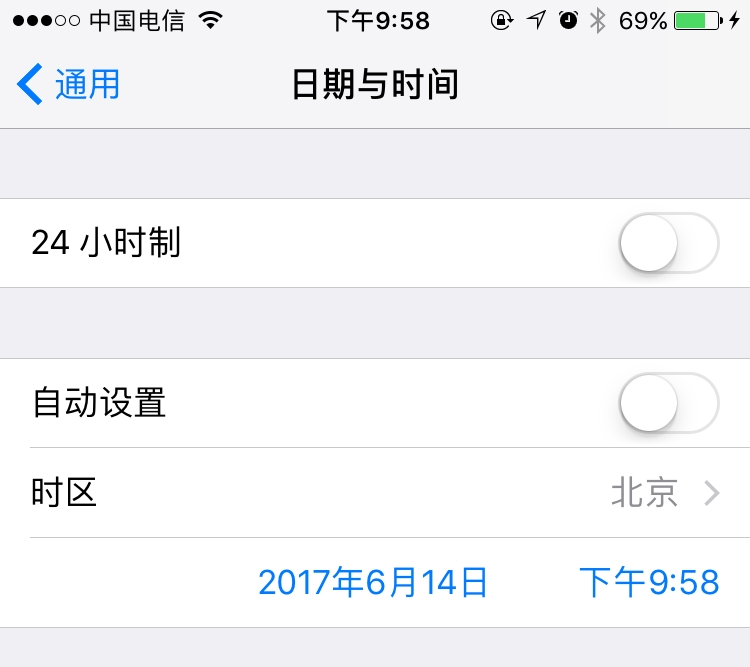#### 第二句话：时区#### 第三句话：时间戳与时区在Code中应用

##### 代码展示
``````package com.vernon.test.time;

import java.text.DateFormat;
import java.text.ParseException;
import java.text.SimpleDateFormat;
import java.util.Calendar;
import java.util.TimeZone;

public class UTCTimecase {

private static DateFormat format = new SimpleDateFormat("yyyy-MM-dd HH:mm");

/**
* 得到UTC时间，类型为字符串，格式为"yyyy-MM-dd HH:mm"<br />
* 如果获取失败，返回null
*
* @return
*/
public static String getUTCTimeStr() {
StringBuffer UTCTimeBuffer = new StringBuffer();
// 1、取得本地时间：
Calendar cal = Calendar.getInstance();
// 2、取得时间偏移量：
int zoneOffset = cal.get(java.util.Calendar.ZONE_OFFSET);
// 3、取得夏令时差：
int dstOffset = cal.get(java.util.Calendar.DST_OFFSET);
// 4、从本地时间里扣除这些差量，即可以取得UTC时间：
int year = cal.get(Calendar.YEAR);
int month = cal.get(Calendar.MONTH) + 1;
int day = cal.get(Calendar.DAY_OF_MONTH);
int hour = cal.get(Calendar.HOUR_OF_DAY);
int minute = cal.get(Calendar.MINUTE);
UTCTimeBuffer.append(year).append("-").append(month).append("-").append(day);
UTCTimeBuffer.append(" ").append(hour).append(":").append(minute);
try {
format.parse(UTCTimeBuffer.toString());
return UTCTimeBuffer.toString();
} catch (ParseException e) {
e.printStackTrace();
}
return null;
}

/**
* 将UTC时间转换为东八区时间
*
* @param UTCTime
* @return
*/
public static String getLocalTimeFromUTC(String UTCTime) {
java.util.Date UTCDate = null;
String localTimeStr = null;
try {
UTCDate = format.parse(UTCTime);
format.setTimeZone(TimeZone.getTimeZone("GMT-8"));
localTimeStr = format.format(UTCDate);
} catch (ParseException e) {
e.printStackTrace();
}

return localTimeStr;
}

public static void main(String[] args) {
String UTCTimeStr = getUTCTimeStr();
System.out.println(UTCTimeStr);
System.out.println(getLocalTimeFromUTC(UTCTimeStr));
}

}
``````
``````package com.vernon.test.time;

import lombok.extern.slf4j.Slf4j;

import java.text.SimpleDateFormat;
import java.util.Calendar;
import java.util.Date;
import java.util.TimeZone;

@Slf4j
public class DateTimeCase {

public static final String FORMAT_DATETIME = "yyyy-MM-dd HH:mm:ss";

public static String getOffsetTime(int offset, Date date) {
TimeZone timeZone = TimeZone.getTimeZone("GMT-" + offset + ":00");
SimpleDateFormat simpleDateFormat = new SimpleDateFormat("yyyy-MM-dd HH:mm:ss");
simpleDateFormat.setTimeZone(timeZone);
return simpleDateFormat.format(date);
}

public static void main(String[] args) {
// 取得本地时间：
Calendar cal1 = Calendar.getInstance();
log.info("cal1={}", new SimpleDateFormat(FORMAT_DATETIME).format(cal1.getTime()));
log.info("cal1={}", cal1.getTime().getTime());

log.info("cal2={}", getOffsetTime(9, cal1.getTime()));
}
}
``````

#### 参考地址